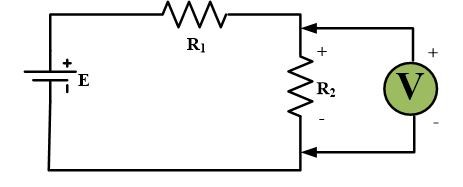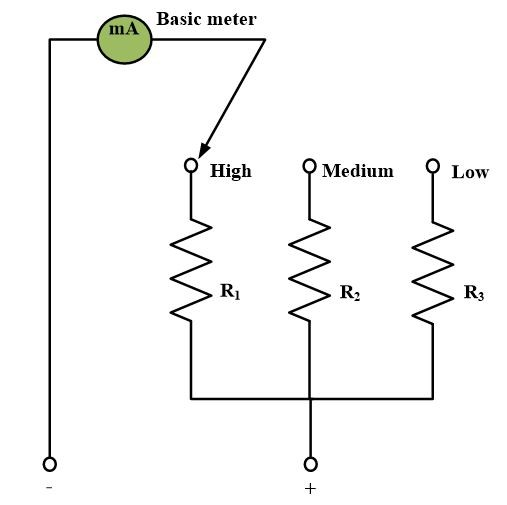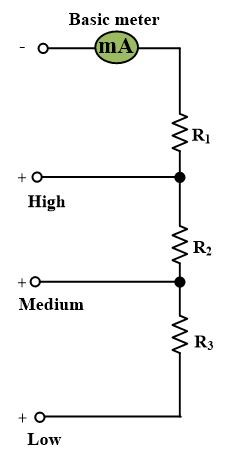Home / Instrumentation / Voltmeter-Definition and Working Principle

# Voltmeter-Definition and Working Principle

Want create site? Find Free WordPress Themes and plugins.

A voltmeter is a basic device used for the measurement of electric potential or voltage, in volts.

As discussed in ammeter section, any basic meter mechanism has a voltage Vm across its terminals when a full-scale current Im, flows through the meter. It follows that basic meter mechanism can be calibrated in micro-volts, milli-volts, or volts, depending on the voltage needed to produce a full-scale deflection. The symbol for the voltmeter is a circle with the enclosed letter V, as indicated in Figure.A voltmeter is connected across (parallel to) the component or a specific portion of a circuit for which the voltage (potential difference) is being measured out.

Just like a DC ammeter, a DC voltmeter also has polarity signs on it; therefore one has to connect the plus (+) terminal of the voltmeter to the higher point of potential and the minus (-) terminal to the lower point of potential in order to obtain an upscale meter deflection.

An AC voltmeter does not have any polarity signs on it, but the instrument is still connected in parallel to the component for which the voltage is desired. As a voltage measurement does not involve breaking the circuit, the voltmeter needs to be connected only when a reading is desired.

A voltmeter with a higher voltage range, V, is constructed by connecting a resistance, Rsc in series with a meter mechanism, having a full-scale voltage capability of Vm as shown in the following Fig.The series resistance is called a multiplier; its value is determined from the voltage equation.

$V={{I}_{m}}{{R}_{se}}+{{V}_{m}}$

Multiple scale voltmeters are constructed either by using switching arrangements or multiple terminals.In above figure, each of the multiplier resistors is separately calculated and are then selected by a non-shorting switch.The multiplier resistors for above figure are calculated by first evaluating the resistance needed for the lowest range. The additional resistance needed for each higher range is then determined.

## Voltage Sensitivity

It is defined as the reciprocal of the current necessary for full-scale deflection.

$Sensitivity=\frac{1}{{{I}_{m}}}~\Omega /Volt$

Where Im is the full-scale current. A smaller meter current results on a larger voltage sensitivity. The actual voltmeter resistance equals the sensitivity times the full-scale voltage. The voltmeter resistance remains constant even through the voltage reading may not be a full-scale reading.

Example

A multi-range voltmeter with 50 and 250 V ranges use a 50 μA meter mechanism.

(a) What is the sensitivity?

(b) What resistance does the voltmeter present n each range?

Solution

(a)

$Sensitivity=\frac{1}{{{I}_{m}}}=\frac{1}{50*{{10}^{-6}}}=20{}^{k\Omega }/{}_{V}$

(b)

$R=sensitivity\text{ }*\text{ }range$

50 V range:

$R=\left( 20*{{10}^{3}} \right)\left( 50 \right)=1*{{10}^{6}}=1~M\Omega$

250 V range:

$R=\left( 20*{{10}^{3}} \right)\left( 250 \right)=5*{{10}^{6}}=5~M\Omega$

Did you find apk for android? You can find new Free Android Games and apps.